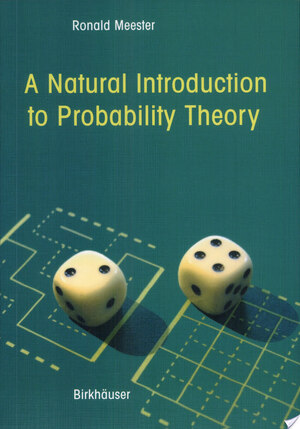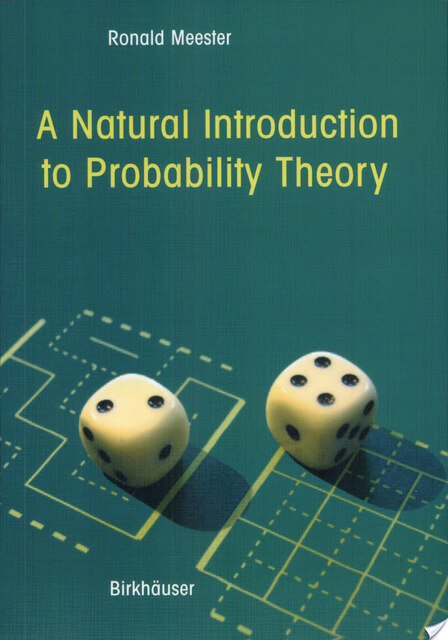Go to top
Voor boeken altijd eerst naar De Slegte

# Boekhandel De Slegte

## Alle vestigingen

Alle vestigingen## Specificaties

• Auteur:
• Uitgever:
Springer Science & Business Media
• ISBN:
9783764321888
• Bindwijze:
Paperback
• Aantal Pagina's:
191
• Genre:
Wetenschap
• Jaar:
2003

# A Natural Introduction to Probability Theory

Beoordeel dit boek als eerste!
Paperback

## Omschrijving

"The book [is] an excellent new introductory text on probability. The classical way of teaching probability is based on measure theory. In this book discrete and continuous probability are studied with mathematical precision, within the realm of Riemann integration and not using notions from measure theorya ]. Numerous topics are discussed, such as: random walks, weak laws of large numbers, infinitely many repetitions, strong laws of large numbers, branching processes, weak convergence and [the] central limit theorem. The theory is illustrated with many original and surprising examples and problems." Zentralblatt Math

"Most textbooks designed for a one-year course in mathematical statistics cover probability in the first few chapters as preparation for the statistics to come. This book in some ways resembles the first part of such textbooks: it's all probability, no statistics. But it does the probability more fully than usual, spending lots of time on motivation, explanation, and rigorous development of the mathematicsa ]. The exposition is usually clear and eloquenta ]. Overall, this is a five-star book on probability that could be used as a textbook or as a supplement." MAA online

Volledige beschrijving

## Verkrijgbaar als Tweedehands

Vestiging
Conditie
Voorraad
Prijs
Laatste exemplaar reeds verkocht.

## Verkrijgbaar als NieuwWeb only

Vestiging
Voorraad
Prijs
Laatste exemplaar reeds verkocht.

## Recensies

### Schrijf een recensie:

Velden met een * zijn verplicht